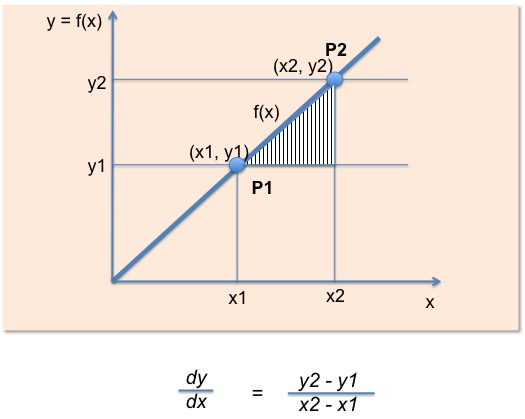# How to Succeed in Math (5 Secrets of Making Math Very Easy) – By a GeniusGood to see you again! Today’s discussion would be Math and I would give you some secrets on how to succeed in Math.

You know, Math have always been and would always be my favorite subject. I learnt it, I practiced it, an I taught it as a teacher some time in the past in secondary school.

So what I’m going to tell you are actually secrets, if you don’t know these five points then you will always likely hate Math. An believe me, Math is actually the most interesting subject.
In this post, I would like to let you know how to succeed in study of Mathematics. I would be talking to you based on my own personal experience.

Point 1: Spend all the Time on it

Assuming that you have been having a particular course, every semester for three years
• MTH 101 – Introduction to Elementary Math

If on the first semester of the fourth year, you have 6 courses with the same Math course included (that is the 7th time you are having this same course), that is Introduction to Mathematics(MTH 101).

The question now is: How easy would this course be at this time?

You guess right?

I tell you, this MTH 101 would be the easiest course for that semester. The reason is that, you’ve spend so much time on a studying Math over the months, therefore it would be the easiest compared to other courses that are totally new. This is in the sense that it would be less difficult.

So What’s the Point?
The point is that the more the percentage of your time you spend in studying Math at the expense of time that would have been used for other causes, the easier Math becomes compared to  those other courses.

This nails the first point.

Spend plenty of time studying Math and you will find out that other courses becomes more difficult(meaning that Math becomes easier!). Even if it appears not interesting, just spend time on it.

I’m sure you get the point now. Let’s move to the the second point.

Point 2: Get a Good(Possibly the Best Textbook)
No matter how anyone may choose to see it, a good textbook would always help you enjoy the study of Math. And the more you enjoy studying Math, the easier it becomes. So don’t just settle with the recommend textbooks in school. Go out there to get the bookshops and get one.
I found out from experience that textbooks written by Americans are always very good when it comes to study of Math. An example is K.A Stroud Engineering Mathematics. If you are in University, no matter the faculty or department, once there is Math in your curriculum, buy Engineering Mathematics.

Point 3: The Principle of First Principles
The principle of First Principles in Mathematics states that, “For you to understand a new topic in Mathematics, learn its first principles”.
Lets take an example: what do you understand by dy/dx in Calculus?This is the meaning of dy/dx from the first principle. The basics is that dy/dx is the slope of the graph. From here you can appreciate how the concept of differentiation and integration, that is the two basic topics in calculus. So what is the point.
To learn a new topic in Math, go all the way to the first principle, and that you can do by finding the first part of the chapter that discusses the topic and the first principles would likely be explained there.

Point 4: Ask For Help From Math Pros (just like me!)

This is very important. As the saying goes that ‘a day with a good teacher is better than a thousand days spend reading a book’. This is so true in the case of Mathematics.
So if there is someone you know that is very good in Mathematics, pester on him to teach you, explain solutions to Math problems, explain the concepts to you an so on. Fortunately, Kindson the Math Pro is there for you. So just paste the Math exercises on the comment box below and I would get back to you.

Point 5: Solve and Solve and Solve!

Get as many examples and exercises as possible. Just keep solving. For textbooks that have solved examples, try to solve it yourself first and then compare your own solution with the example in the textbook. For the exercises, solve all if you can and then verify if your answer is correct.
I assure you that I you apply this five points, you will make so much improvement in Math and with time you will find Math very interesting. Trust me!

I will stop here for now, and I would like to thank you for reading. If this have been informative for your tell me how you feel in the comment box below and Follow me on Google+.
You can as wells subscribe to my YouTube channel at Kindson The Math Pro on YouTube